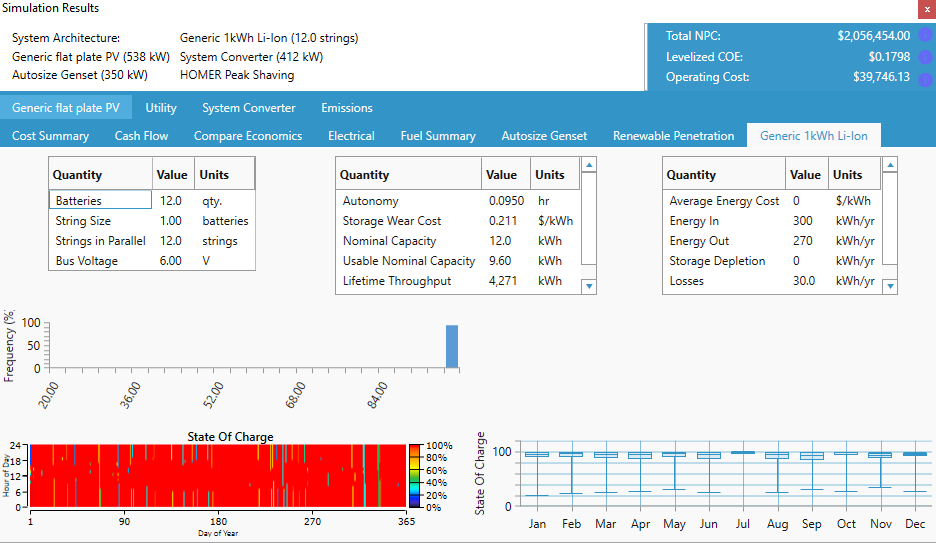# HOMER Grid 1.4

 Navigation: Results > Tables Mode > Simulation Results Storage OutputsThe Storage tab in the Simulation Results window contains the following output variables.

 Variable Description Batteries The number of batteries in the array is the string size multiplied by the number of strings String Size The number of batteries connected in series in each string Strings in Parallel The number of storage strings connected in parallel Bus Voltage The voltage of the storage array, calculated by multiplying storage voltage by string size, in volts Autonomy The capacity of the storage bank divided by the average electrical load, in hours Storage Wear Cost The cost of cycling energy through the storage bank, in \$/kWh Nominal Capacity The amount of energy that could be withdrawn from the storage at a particular constant current, starting from a fully charged state, in kWh Usable Nominal Capacity The storage capacity adjusted to exclude all capacity below the minimum state of charge of the storage, in kWh Lifetime Throughput The total amount of energy that can be cycled through the storage before it needs to be replaced, in kWh Expected Life The number of years the storage bank will last before it requires replacement Average Energy Cost The average cost of the energy that goes into the storage, in \$/kWh Energy In The total amount of energy charged to the storage, in kWh Energy Out The total amount of energy discharged from the storage, in kWh Storage Depletion The difference in the storage state of charge at the beginning and end of the year, in kWh/yr Losses Annual energy losses due to storage inefficiency, in kWh/yr Annual Throughput The total amount of energy that cycled through the storage bank during the year, in kWh/yr

In the bottom half of the page a DMap appears showing the state of charge of the storage bank in each time step of the year. Below is an example of Storage output in HOMER Grid.## Time Series Outputs

You can click the Plot button in the Time Series Output window to view a plot of system operation time-step-by-time-step.

 Variable Description Maximum Charge Power The maximum power (kW) that the battery can be charged in each time step. The actual power output is less than or equal to this amount. Maximum Discharge Power The maximum power (kW) that the battery can supply (the actual power output is less than or equal to this amount). The battery contributes this amount to the operating capacity. Charge Power The charge power (kW) in each time step. The charge power is zero if the battery is being discharged. Discharge Power The output power (kW) that the battery supplies. The discharge power is zero if the battery is being charged. Input Power The power flow (kW) in or out of the battery. Positive is charging, negative is discharging. This is equivalent to the Charge Power when the battery is charging, and to the negative of the Discharge Power when the battery is discharging. Energy Content The total energy currently stored in the battery, in kWh. State of Charge The energy currently stored in the battery, as a percentage of the maximum capacity of the battery. Energy Cost The average marginal cost of energy added to the storage bank in cost per kWh. This value is used in dispatch decisions. Temperature The internal temperature of the battery in Celsius (Modified Kinetic Battery model only). Degradation - Time and Temperature The degradation of the battery due to age and temperature history. This value works with the Degradation-Cycling value to determine the performance degradation of the battery and replacement time (Modified Kinetic Battery model only). Degradation - Cycling The degradation of the battery due to use. This value works with the value Degradation - Time and Temperature to determine the performance degradation of the battery and replacement time (Modified Kinetic Battery model only). Equivalent Cycles The cumulative count of total equivalent full cycles of the battery computed with the Rainflow counting algorithm (Modified Kinetic Battery model only).

The Homer Support site has a searchable knowledgebase and additional support options. HOMER Online contains the latest information on model updates, as well as sample files, resource data, and contact information. ©2017 HOMER Energy, LLC